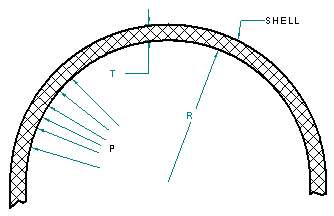### Pressure Vessel Wall Thickness with Internal Pressure Applied Calculator

Pressure Vessels Design and Engineering

This calculator determines the thickness of a circumferential shell with an internal pressure applied: Typical applications are propane tanks, compressed air storage tanks etc.

The "Joint Efficiency" reflects your confidence in the welded seam areas of the as-manufactured pressure vessel.Thickness of Circumferential Shells Under Internal Pressure: Design Variables: (P) = Design Pressure (internal) (psi) = (R) = Inside radius of shell under consideration (in.) = (S) = Max. Allowable Stress Material (psi) = (E) Joint Efficiency (80% = .80) = Result: Minimum required wall thickness of shell (inches) =